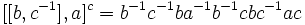# Witt's identity

This fact is related to: commutator calculus
View other facts related to commutator calculusView terms related to commutator calculus |

## Statement

### In terms of right-action convention

Let$a,b,c$ be elements of an arbitrary group$G$. Then:$\! [[a,b^{-1}],c]^b \cdot [[b,c^{-1}],a]^c \cdot [[c,a^{-1}],b]^a = e$

where$[x,y] = x^{-1}y^{-1}xy$ and$x^y = y^{-1}xy$, and$e$ is the identity element of the group.

## Proof

### In terms of right-action convention

Given: A group$G$, elements$a,b,c \in G$.$e$ is the identity element.

To prove:$\! [[a,b^{-1}],c]^b \cdot [[b,c^{-1}],a]^c \cdot [[c,a^{-1}],b]^a = e$ where$[x,y] := x^{-1}y^{-1}xy$ and$x^y = y^{-1}xy$.

Proof: We start out with the first term on the left side:$\! [[a,b^{-1}],c]^b = [a^{-1}bab^{-1},c]^b = b^{-1}[a^{-1}bab^{-1},c]b = b^{-1}ba^{-1}b^{-1}ac^{-1}a^{-1}bab^{-1}cb = a^{-1}b^{-1}ac^{-1}a^{-1}bab^{-1}cb$

Similarly, we have:$\! [[b,c^{-1}],a]^c = b^{-1}c^{-1}ba^{-1}b^{-1}cbc^{-1}ac$

and:$\! [[c,a^{-1}],b]^a = c^{-1}a^{-1}cb^{-1}c^{-1}aca^{-1}ba$

Multiplying these, all terms cancel and we obtain the identity element, as desired.